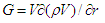# Gravitation and gravitational waves

Let’s demonstrate that the creation of any elementary particle is accompanied by appearance of the gravitation, notably the pressure force in physical vacuum, generated by small periodic perturbations of its density, which in its own turn generate gravitational wave, propagating to the center of newborn particle. It’s natural to propose that gravitation works over any distance from the particle, and that when the distance is large, perturbations of physical vacuum density created by the newborn particle depend only on distance r and are independent of angles θ and φ . With such assumption, let’s seek solutions of equations system of elementary particles when r is large in the following form:Then we shall obtain the system of equations for gravitation waves in the form ofmeaning that of all four fields in the initial equations system of elementary particles only gravitational fieldwill remain significant when r is large enough.

Furthermore, gravitational field differs from three other fields considered at analysis of elementary particles since it’s severely nonlinear field. It can’t be linearized basing on the form of velocity W component in analogue with electric and two internal fields of the particle. When r is small and, consequentially, V is small as well, gravitational field can be neglected during the formulation of elementary particles theory (though, possibly, when r, exactly gravitational field describes also nuclear interactions). On the contrary, when r is relatively large, all other fields with the exception of gravitational can be neglected, and that agrees with experimental data

Let’s seek the solution of equations system for gravitational waves in the form of V = c/r2, that is in the form of a wave, which propagates to the center of elementary particle (r = 0) with a velocity dependant on radius. Then the next expression will be derived for density of physical vacuum in the case of r→∞The pressure force of gravitational wave (gravitational field intensity) expresses asand it agrees with the law of universal gravitation. However, the physical essence of gravitation comes in somewhat different light than before. The bodies do not attract each other – each material body creates its own gravitational wave, which propagates from infinity to its center of mass and puts an external pressure on other body with the force, proportional to the mass of the body and inversely proportional to the square of distance between the bodies.

Let’s note another significant difference between gravitational and electromagnetic waves. Electromagnetic wave moving with constant velocity has a wavelength, thus, resulting in the existence of electromagnetic wave quant or photon. Gravitational wave moves with velocity dependant on radius, thus, there can be no such thing as gravitational wave quant. Traditional parallel between the gravitational wave and its hypothetical carrier, graviton, is apparently the main obstacle for the real discovery of gravitational waves in nature.